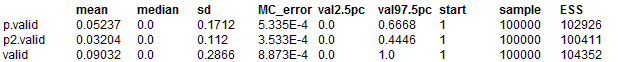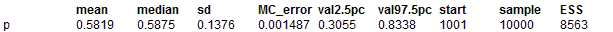#### Coins: the ABC of coin tossing

Suppose that a coin is tossed ten time and six of those tosses come up heads. What does this information say about the probability of the coin comming up heads if tossed?

We can model a coin toss as a draw from a bernoulli distribution with proportion p:

r ~ Bernoulli(p)

with the convention that a value of r equal to one corresponds to heads. The proportion p is unkown but we know it must lie between zero and one so we can model it as

p ~ Uniform(0, 1)

We can model our coin tossing experiment by simulating a p from the uniform distribution and then simulating ten bernoulli deviates using this value of p. This is a forward sampling simulation and is easily carried out with the BUGS software, the model is

model{
p ~ dunif(0, 1)
for (i in 1 : 10){
r[i] ~ dbern(p)
}
}

When we run this model not all the updates will lead to exactly six heads. So we need to add a bit more code to the model to pick out the cases where there are exactly six heads.

model{
p ~ dunif(0, 1)
for (i in 1 : 10){
r[i] ~ dbern(p)
}
r.total <- sum(r[]);
valid <- equals(r.total, 6)
p.valid <- p * valid
p2.valid <- p * p * valid
}

We are only interested in the updates that have r.total equal to six. For these updates valid is one and for the other updates valid is zero, so for valid equal to one p.valid will equal p and for valid equal to zero p.valid will equal zero. We can calculate the mean value of p for those updates for which r.total equals six by dividing the mean of p.valid by the mean of valid. We can in the same way calculate the mean of p squared using p2.valid and hence the standard deviation of p for r.total equal to six.

ResultsMean value of p is estimated as 0.5798, mean value of p squared as 0.3547 and the sd of p as 0.1361. Note that there are eleven possible values of r.total and they are each equally likely then we would expect the mean of valid to be 1 / 11 which is close to what we see.

This technique of sampling the model with out taking into account any observations and then
picking out only those realizations that correspond to the observations is called Approximate Bayesian Computions or ABC. For our simple coin tossing model we can easily write down the Bayesian model:

model{
p ~ dunif(0, 1)
r ~ dbin(p, 10)
r <- 6
}

Running this model in BUGS gives the following estimate of p